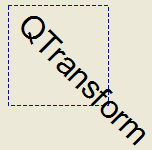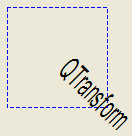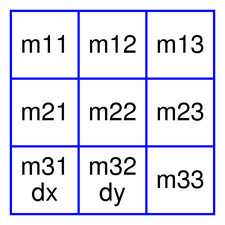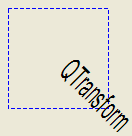# QTransform¶

The `QTransform` class specifies 2D transformations of a coordinate system. More## Detailed Description¶

A transformation specifies how to translate, scale, shear, rotate or project the coordinate system, and is typically used when rendering graphics.

`QTransform` differs from `QMatrix` in that it is a true 3x3 matrix, allowing perspective transformations. `QTransform` ‘s `toAffine()` method allows casting `QTransform` to `QMatrix` . If a perspective transformation has been specified on the matrix, then the conversion will cause loss of data.

`QTransform` is the recommended transformation class in Qt.

A `QTransform` object can be built using the `setMatrix()` , `scale()` , `rotate()` , `translate()` and `shear()` functions. Alternatively, it can be built by applying `basic matrix operations` . The matrix can also be defined when constructed, and it can be reset to the identity matrix (the default) using the `reset()` function.

The `QTransform` class supports mapping of graphic primitives: A given point, line, polygon, region, or painter path can be mapped to the coordinate system defined by this matrix using the `map()` function. In case of a rectangle, its coordinates can be transformed using the `mapRect()` function. A rectangle can also be transformed into a polygon (mapped to the coordinate system defined by this matrix), using the `mapToPolygon()` function.

`QTransform` provides the `isIdentity()` function which returns `true` if the matrix is the identity matrix, and the `isInvertible()` function which returns `true` if the matrix is non-singular (i.e. AB = BA = I). The `inverted()` function returns an inverted copy of this matrix if it is invertible (otherwise it returns the identity matrix), and `adjoint()` returns the matrix’s classical adjoint. In addition, `QTransform` provides the `determinant()` function which returns the matrix’s determinant.

Finally, the `QTransform` class supports matrix multiplication, addition and subtraction, and objects of the class can be streamed as well as compared.

### Rendering Graphics¶

When rendering graphics, the matrix defines the transformations but the actual transformation is performed by the drawing routines in `QPainter` .

By default, `QPainter` operates on the associated device’s own coordinate system. The standard coordinate system of a `QPaintDevice` has its origin located at the top-left position. The x values increase to the right; y values increase downward. For a complete description, see the coordinate system documentation.

`QPainter` has functions to translate, scale, shear and rotate the coordinate system without using a `QTransform` . For example:```def paintEvent(self, event) painter = QPainter(self) painter.setPen(QPen(Qt.blue, 1, Qt.DashLine)) painter.drawRect(0, 0, 100, 100) painter.rotate(45) painter.setFont(QFont("Helvetica", 24)) painter.setPen(QPen(Qt.black, 1)) painter.drawText(20, 10, "QTransform") ```

Although these functions are very convenient, it can be more efficient to build a `QTransform` and call `setTransform()` if you want to perform more than a single transform operation. For example:```def paintEvent(self, event) painter = QPainter(self) painter.setPen(QPen(Qt.blue, 1, Qt.DashLine)) painter.drawRect(0, 0, 100, 100) transform = QTransform() transform.translate(50, 50) transform.rotate(45) transform.scale(0.5, 1.0) painter.setTransform(transform) painter.setFont(QFont("Helvetica", 24)) painter.setPen(QPen(Qt.black, 1)) painter.drawText(20, 10, "QTransform") ```

### Basic Matrix Operations¶A `QTransform` object contains a 3 x 3 matrix. The `m31` (`dx` ) and `m32` (`dy` ) elements specify horizontal and vertical translation. The `m11` and `m22` elements specify horizontal and vertical scaling. The `m21` and `m12` elements specify horizontal and vertical shearing . And finally, the `m13` and `m23` elements specify horizontal and vertical projection, with `m33` as an additional projection factor.

`QTransform` transforms a point in the plane to another point using the following formulas:

```x' = m11*x + m21*y + dx
y' = m22*y + m12*x + dy
if is not affine:
w' = m13*x + m23*y + m33
x' /= w'
y' /= w'
```

The point (x, y) is the original point, and (x’, y’) is the transformed point. (x’, y’) can be transformed back to (x, y) by performing the same operation on the `inverted()` matrix.

The various matrix elements can be set when constructing the matrix, or by using the `setMatrix()` function later on. They can also be manipulated using the `translate()` , `rotate()` , `scale()` and `shear()` convenience functions. The currently set values can be retrieved using the `m11()` , `m12()` , `m13()` , `m21()` , `m22()` , `m23()` , `m31()` , `m32()` , `m33()` , `dx()` and `dy()` functions.

Translation is the simplest transformation. Setting `dx` and `dy` will move the coordinate system `dx` units along the X axis and `dy` units along the Y axis. Scaling can be done by setting `m11` and `m22` . For example, setting `m11` to 2 and `m22` to 1.5 will double the height and increase the width by 50%. The identity matrix has `m11` , `m22` , and `m33` set to 1 (all others are set to 0) mapping a point to itself. Shearing is controlled by `m12` and `m21` . Setting these elements to values different from zero will twist the coordinate system. Rotation is achieved by setting both the shearing factors and the scaling factors. Perspective transformation is achieved by setting both the projection factors and the scaling factors.

Here’s the combined transformations example using basic matrix operations:```def paintEvent(self, event) pi = 3.14 a = pi/180 * 45.0 sina = sin(a) cosa = cos(a) translationTransform = QTransform(1, 0, 0, 1, 50.0, 50.0) rotationTransform = QTransform(cosa, sina, -sina, cosa, 0, 0) scalingTransform = QTransform(0.5, 0, 0, 1.0, 0, 0) transform = QTransform() transform = scalingTransform * rotationTransform * translationTransform painter = QPainter(self) painter.setPen(QPen(Qt.blue, 1, Qt.DashLine)) painter.drawRect(0, 0, 100, 100) painter.setTransform(transform) painter.setFont(QFont("Helvetica", 24)) painter.setPen(QPen(Qt.black, 1)) painter.drawText(20, 10, "QTransform") ```
class `QTransform`

QTransform(mtx)

QTransform(other)

QTransform(h11, h12, h13, h21, h22, h23, h31, h32[, h33=1.0])

QTransform(h11, h12, h21, h22, dx, dy)

param h32

`qreal`

param h33

`qreal`

param h21

`qreal`

param h22

`qreal`

param h23

`qreal`

param mtx

`QMatrix`

param h11

`qreal`

param h12

`qreal`

param h13

`qreal`

param dx

`qreal`

param dy

`qreal`

param other

`QTransform`

param h31

`qreal`

Constructs an identity matrix.

All elements are set to zero except `m11` and `m22` (specifying the scale) and `m33` which are set to 1.

`reset()`

Constructs a matrix with the elements, `m11` , `m12` , `m13` , `m21` , `m22` , `m23` , `m31` , `m32` , `m33` .

`setMatrix()`

Constructs a matrix with the elements, `m11` , `m12` , `m21` , `m22` , `dx` and `dy` .

`setMatrix()`

`PySide2.QtGui.QTransform.``TransformationType`

Constant

Description

QTransform.TxNone

QTransform.TxTranslate

QTransform.TxScale

QTransform.TxRotate

QTransform.TxShear

QTransform.TxProject

`PySide2.QtGui.QTransform.``__reduce__`()
Return type

`PyObject`

`PySide2.QtGui.QTransform.``__repr__`()
Return type

`PyObject`

`PySide2.QtGui.QTransform.``adjoint`()
Return type

`QTransform`

Returns the adjoint of this matrix.

`PySide2.QtGui.QTransform.``det`()
Return type

`qreal`

Note

This function is deprecated.

Returns the matrix’s determinant. Use `determinant()` instead.

`PySide2.QtGui.QTransform.``determinant`()
Return type

`qreal`

Returns the matrix’s determinant.

`PySide2.QtGui.QTransform.``dx`()
Return type

`qreal`

Returns the horizontal translation factor.

`m31()` `translate()` `Basic Matrix Operations`

`PySide2.QtGui.QTransform.``dy`()
Return type

`qreal`

Returns the vertical translation factor.

`translate()` `Basic Matrix Operations`

static `PySide2.QtGui.QTransform.``fromScale`(dx, dy)
Parameters
• dx`qreal`

• dy`qreal`

Return type

`QTransform`

Creates a matrix which corresponds to a scaling of `sx` horizontally and `sy` vertically. This is the same as `QTransform()` .scale(sx, sy) but slightly faster.

static `PySide2.QtGui.QTransform.``fromTranslate`(dx, dy)
Parameters
• dx`qreal`

• dy`qreal`

Return type

`QTransform`

Creates a matrix which corresponds to a translation of `dx` along the x axis and `dy` along the y axis. This is the same as `QTransform()` .translate(dx, dy) but slightly faster.

`PySide2.QtGui.QTransform.``inverted`()
Return type

PyTuple

Returns an inverted copy of this matrix.

If the matrix is singular (not invertible), the returned matrix is the identity matrix. If `invertible` is valid (i.e. not 0), its value is set to true if the matrix is invertible, otherwise it is set to false.

`isInvertible()`

`PySide2.QtGui.QTransform.``isAffine`()
Return type

`bool`

Returns `true` if the matrix represent an affine transformation, otherwise returns `false` .

`PySide2.QtGui.QTransform.``isIdentity`()
Return type

`bool`

Returns `true` if the matrix is the identity matrix, otherwise returns `false` .

`reset()`

`PySide2.QtGui.QTransform.``isInvertible`()
Return type

`bool`

Returns `true` if the matrix is invertible, otherwise returns `false` .

`inverted()`

`PySide2.QtGui.QTransform.``isRotating`()
Return type

`bool`

Returns `true` if the matrix represents some kind of a rotating transformation, otherwise returns `false` .

Note

A rotation transformation of 180 degrees and/or 360 degrees is treated as a scaling transformation.

`reset()`

`PySide2.QtGui.QTransform.``isScaling`()
Return type

`bool`

Returns `true` if the matrix represents a scaling transformation, otherwise returns `false` .

`reset()`

`PySide2.QtGui.QTransform.``isTranslating`()
Return type

`bool`

Returns `true` if the matrix represents a translating transformation, otherwise returns `false` .

`reset()`

`PySide2.QtGui.QTransform.``m11`()
Return type

`qreal`

Returns the horizontal scaling factor.

`scale()` `Basic Matrix Operations`

`PySide2.QtGui.QTransform.``m12`()
Return type

`qreal`

Returns the vertical shearing factor.

`shear()` `Basic Matrix Operations`

`PySide2.QtGui.QTransform.``m13`()
Return type

`qreal`

Returns the horizontal projection factor.

`translate()` `Basic Matrix Operations`

`PySide2.QtGui.QTransform.``m21`()
Return type

`qreal`

Returns the horizontal shearing factor.

`shear()` `Basic Matrix Operations`

`PySide2.QtGui.QTransform.``m22`()
Return type

`qreal`

Returns the vertical scaling factor.

`scale()` `Basic Matrix Operations`

`PySide2.QtGui.QTransform.``m23`()
Return type

`qreal`

Returns the vertical projection factor.

`translate()` `Basic Matrix Operations`

`PySide2.QtGui.QTransform.``m31`()
Return type

`qreal`

Returns the horizontal translation factor.

`dx()` `translate()` `Basic Matrix Operations`

`PySide2.QtGui.QTransform.``m32`()
Return type

`qreal`

Returns the vertical translation factor.

`dy()` `translate()` `Basic Matrix Operations`

`PySide2.QtGui.QTransform.``m33`()
Return type

`qreal`

Returns the division factor.

`translate()` `Basic Matrix Operations`

`PySide2.QtGui.QTransform.``map`(x, y)
Parameters
• x`qreal`

• y`qreal`

Maps the given coordinates `x` and `y` into the coordinate system defined by this matrix. The resulting values are put in *``tx`` and *``ty`` , respectively.

The coordinates are transformed using the following formulas:

```x' = m11*x + m21*y + dx
y' = m22*y + m12*x + dy
if is not affine:
w' = m13*x + m23*y + m33
x' /= w'
y' /= w'
```

The point (x, y) is the original point, and (x’, y’) is the transformed point.

`Basic Matrix Operations`

`PySide2.QtGui.QTransform.``map`(r)
Parameters
Return type

`QRegion`

`PySide2.QtGui.QTransform.``map`(a)
Parameters
Return type

`QPolygonF`

`PySide2.QtGui.QTransform.``map`(a)
Parameters
Return type

`QPolygon`

`PySide2.QtGui.QTransform.``map`(p)
Parameters

p`QPoint`

Return type

`QPoint`

`PySide2.QtGui.QTransform.``map`(p)
Parameters

p`QPointF`

Return type

`QPointF`

`PySide2.QtGui.QTransform.``map`(l)
Parameters

l`QLine`

Return type

`QLine`

`PySide2.QtGui.QTransform.``map`(p)
Parameters
Return type

`QPainterPath`

`PySide2.QtGui.QTransform.``map`(l)
Parameters

l`QLineF`

Return type

`QLineF`

`PySide2.QtGui.QTransform.``mapRect`(arg__1)
Parameters

arg__1`QRect`

Return type

`QRect`

`PySide2.QtGui.QTransform.``mapRect`(arg__1)
Parameters

arg__1`QRectF`

Return type

`QRectF`

`PySide2.QtGui.QTransform.``mapToPolygon`(r)
Parameters

r`QRect`

Return type

`QPolygon`

Creates and returns a `QPolygon` representation of the given `rectangle` , mapped into the coordinate system defined by this matrix.

The rectangle’s coordinates are transformed using the following formulas:

```x' = m11*x + m21*y + dx
y' = m22*y + m12*x + dy
if is not affine:
w' = m13*x + m23*y + m33
x' /= w'
y' /= w'
```

Polygons and rectangles behave slightly differently when transformed (due to integer rounding), so `matrix.map(QPolygon(rectangle))` is not always the same as `matrix.mapToPolygon(rectangle)` .

`mapRect()` `Basic Matrix Operations`

`PySide2.QtGui.QTransform.``__ne__`(arg__1)
Parameters

arg__1`QTransform`

Return type

`bool`

Returns `true` if this matrix is not equal to the given `matrix` , otherwise returns `false` .

`PySide2.QtGui.QTransform.``__mul__`(n)
Parameters

n`qreal`

Return type

`QTransform`

`PySide2.QtGui.QTransform.``__mul__`(o)
Parameters
Return type

`QTransform`

Returns the result of multiplying this matrix by the given `matrix` .

Note that matrix multiplication is not commutative, i.e. a*b != b*a.

`PySide2.QtGui.QTransform.``__imul__`(arg__1)
Parameters

arg__1`QTransform`

Return type

`QTransform`

`PySide2.QtGui.QTransform.``__imul__`(div)
Parameters

div`qreal`

Return type

`QTransform`

Returns the result of performing an element-wise multiplication of this matrix with the given `scalar` .

`PySide2.QtGui.QTransform.``__add__`(n)
Parameters

n`qreal`

Return type

`QTransform`

`PySide2.QtGui.QTransform.``__iadd__`(div)
Parameters

div`qreal`

Return type

`QTransform`

Returns the matrix obtained by adding the given `scalar` to each element of this matrix.

`PySide2.QtGui.QTransform.``__sub__`(n)
Parameters

n`qreal`

Return type

`QTransform`

`PySide2.QtGui.QTransform.``__isub__`(div)
Parameters

div`qreal`

Return type

`QTransform`

Returns the matrix obtained by subtracting the given `scalar` from each element of this matrix.

`PySide2.QtGui.QTransform.``__div__`(n)
Parameters

n`qreal`

Return type

`QTransform`

`PySide2.QtGui.QTransform.``__idiv__`(div)
Parameters

div`qreal`

Return type

`QTransform`

Returns the result of performing an element-wise division of this matrix by the given `scalar` .

`PySide2.QtGui.QTransform.``__eq__`(arg__1)
Parameters

arg__1`QTransform`

Return type

`bool`

Returns `true` if this matrix is equal to the given `matrix` , otherwise returns `false` .

static `PySide2.QtGui.QTransform.``quadToQuad`(arg__1, arg__2)
Parameters
Return type

`PyObject`

static `PySide2.QtGui.QTransform.``quadToQuad`(one, two, result)
Parameters
Return type

`bool`

Creates a transformation matrix, `trans` , that maps a four-sided polygon, `one` , to another four-sided polygon, `two` . Returns `true` if the transformation is possible; otherwise returns false.

This is a convenience method combining `quadToSquare()` and `squareToQuad()` methods. It allows the input quad to be transformed into any other quad.

`squareToQuad()` `quadToSquare()`

static `PySide2.QtGui.QTransform.``quadToSquare`(arg__1)
Parameters

arg__1`QPolygonF`

Return type

`PyObject`

static `PySide2.QtGui.QTransform.``quadToSquare`(quad, result)
Parameters
Return type

`bool`

Creates a transformation matrix, `trans` , that maps a four-sided polygon, `quad` , to a unit square. Returns `true` if the transformation is constructed or false if such a transformation does not exist.

`squareToQuad()` `quadToQuad()`

`PySide2.QtGui.QTransform.``reset`()

Resets the matrix to an identity matrix, i.e. all elements are set to zero, except `m11` and `m22` (specifying the scale) and `m33` which are set to 1.

`QTransform()` `isIdentity()` `Basic Matrix Operations`

`PySide2.QtGui.QTransform.``rotate`(a[, axis=Qt.ZAxis])
Parameters
• a`qreal`

• axis`Axis`

Return type

`QTransform`

Rotates the coordinate system counterclockwise by the given `angle` about the specified `axis` and returns a reference to the matrix.

Note that if you apply a `QTransform` to a point defined in widget coordinates, the direction of the rotation will be clockwise because the y-axis points downwards.

The angle is specified in degrees.

`setMatrix()`

`PySide2.QtGui.QTransform.``rotateRadians`(a[, axis=Qt.ZAxis])
Parameters
• a`qreal`

• axis`Axis`

Return type

`QTransform`

Rotates the coordinate system counterclockwise by the given `angle` about the specified `axis` and returns a reference to the matrix.

Note that if you apply a `QTransform` to a point defined in widget coordinates, the direction of the rotation will be clockwise because the y-axis points downwards.

The angle is specified in radians.

`setMatrix()`

`PySide2.QtGui.QTransform.``scale`(sx, sy)
Parameters
• sx`qreal`

• sy`qreal`

Return type

`QTransform`

Scales the coordinate system by `sx` horizontally and `sy` vertically, and returns a reference to the matrix.

`setMatrix()`

`PySide2.QtGui.QTransform.``setMatrix`(m11, m12, m13, m21, m22, m23, m31, m32, m33)
Parameters
• m11`qreal`

• m12`qreal`

• m13`qreal`

• m21`qreal`

• m22`qreal`

• m23`qreal`

• m31`qreal`

• m32`qreal`

• m33`qreal`

Sets the matrix elements to the specified values, `m11` , `m12` , `m13` `m21` , `m22` , `m23` `m31` , `m32` and `m33` . Note that this function replaces the previous values. `QTransform` provides the `translate()` , `rotate()` , `scale()` and `shear()` convenience functions to manipulate the various matrix elements based on the currently defined coordinate system.

`QTransform()`

`PySide2.QtGui.QTransform.``shear`(sh, sv)
Parameters
• sh`qreal`

• sv`qreal`

Return type

`QTransform`

Shears the coordinate system by `sh` horizontally and `sv` vertically, and returns a reference to the matrix.

`setMatrix()`

static `PySide2.QtGui.QTransform.``squareToQuad`(arg__1)
Parameters

arg__1`QPolygonF`

Return type

`PyObject`

static `PySide2.QtGui.QTransform.``squareToQuad`(square, result)
Parameters
Return type

`bool`

Creates a transformation matrix, `trans` , that maps a unit square to a four-sided polygon, `quad` . Returns `true` if the transformation is constructed or false if such a transformation does not exist.

`quadToSquare()` `quadToQuad()`

`PySide2.QtGui.QTransform.``toAffine`()
Return type

`QMatrix`

Returns the `QTransform` as an affine matrix.

Warning

If a perspective transformation has been specified, then the conversion will cause loss of data.

`PySide2.QtGui.QTransform.``translate`(dx, dy)
Parameters
• dx`qreal`

• dy`qreal`

Return type

`QTransform`

Moves the coordinate system `dx` along the x axis and `dy` along the y axis, and returns a reference to the matrix.

`setMatrix()`

`PySide2.QtGui.QTransform.``transposed`()
Return type

`QTransform`

Returns the transpose of this matrix.

`PySide2.QtGui.QTransform.``type`()
Return type

`TransformationType`

Returns the transformation type of this matrix.

The transformation type is the highest enumeration value capturing all of the matrix’s transformations. For example, if the matrix both scales and shears, the type would be `TxShear` , because `TxShear` has a higher enumeration value than `TxScale` .

Knowing the transformation type of a matrix is useful for optimization: you can often handle specific types more optimally than handling the generic case.